# Weierstrass conditions (for a variational extremum)

(diff) ← Older revision | Latest revision (diff) | Newer revision → (diff)

Necessary and (partially) sufficient conditions for a strong extremum in the classical calculus of variations (cf. Variational calculus). Proposed in 1879 by K. Weierstrass.

Weierstrass' necessary condition: For the functional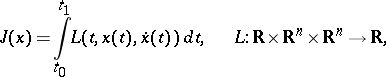to attain a strong local minimum on the extremal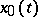, it is necessary that the inequality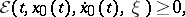where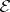is the Weierstrass-function, be satisfied for all,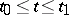, and all. This condition may be expressed in terms of the function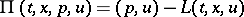(cf. Pontryagin maximum principle). The Weierstrass condition (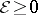on the extremal) is equivalent to saying that the function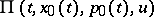, where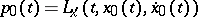, attains a maximum infor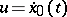. Thus, Weierstrass' necessary condition is a special case of the Pontryagin maximum principle.

Weierstrass' sufficient condition: For the functionalto attain a strong local minimum on the vector function, it is sufficient that there exists a vector-valued field slope function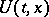(geodesic slope) (cf. Hilbert invariant integral) in a neighbourhoodof the curve, for which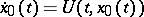and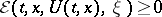for all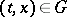and any vector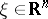.

How to Cite This Entry:
Weierstrass conditions (for a variational extremum). Encyclopedia of Mathematics. URL: http://encyclopediaofmath.org/index.php?title=Weierstrass_conditions_(for_a_variational_extremum)&oldid=17563
This article was adapted from an original article by V.M. Tikhomirov (originator), which appeared in Encyclopedia of Mathematics - ISBN 1402006098. See original article